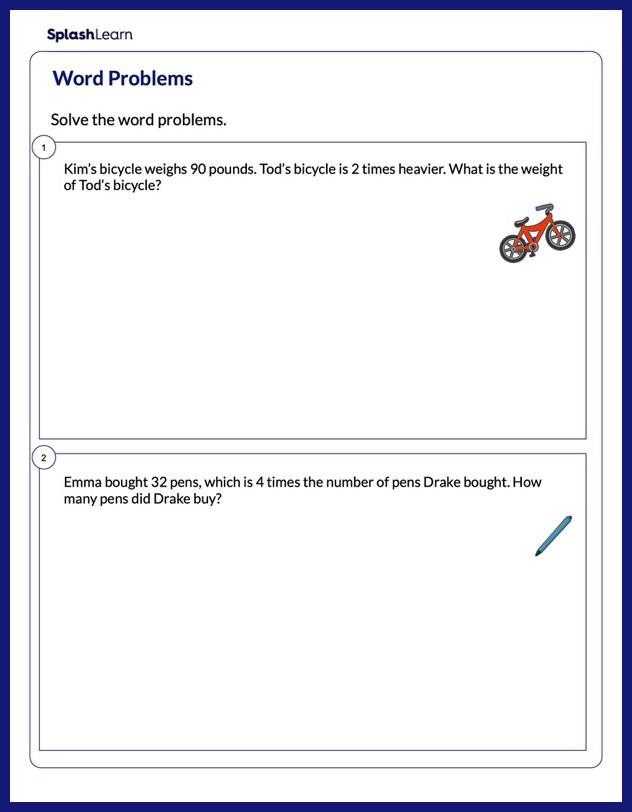# Solve the Multiplication Word Problems Worksheet

Home > Solve the Multiplication Word ProblemsImprove your child's proficiency in multiplication with this worksheet. Young learners will make connections between math and the real world as they solve a set of multiplication word problems involving multiply by scenarios. In these problems, they comprehend the scenarios and find the unknown quantity. This worksheet will nudge your young mathematician toward mastery and develop their all round mathematical acumen.